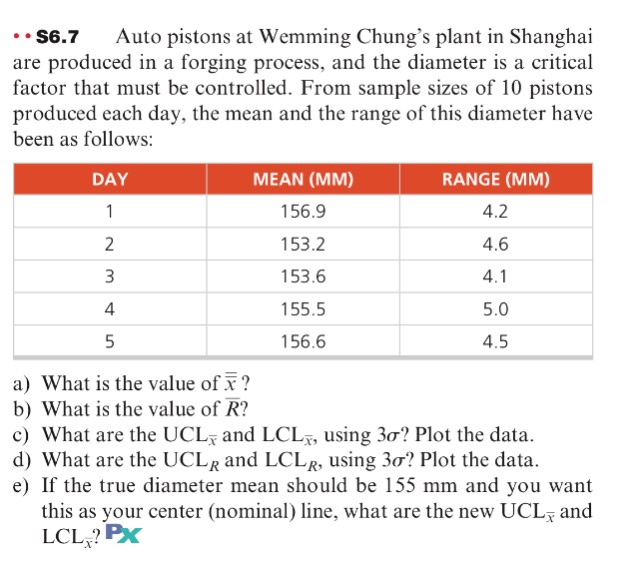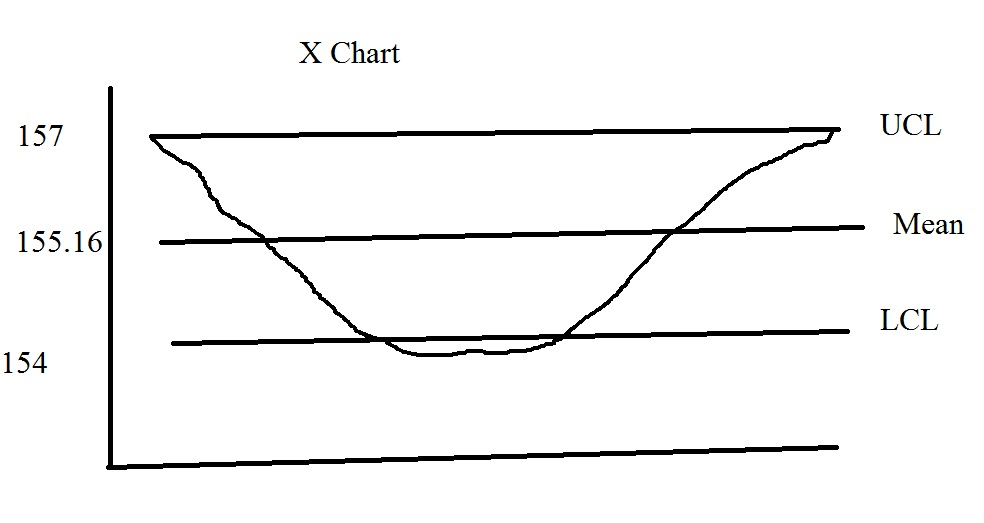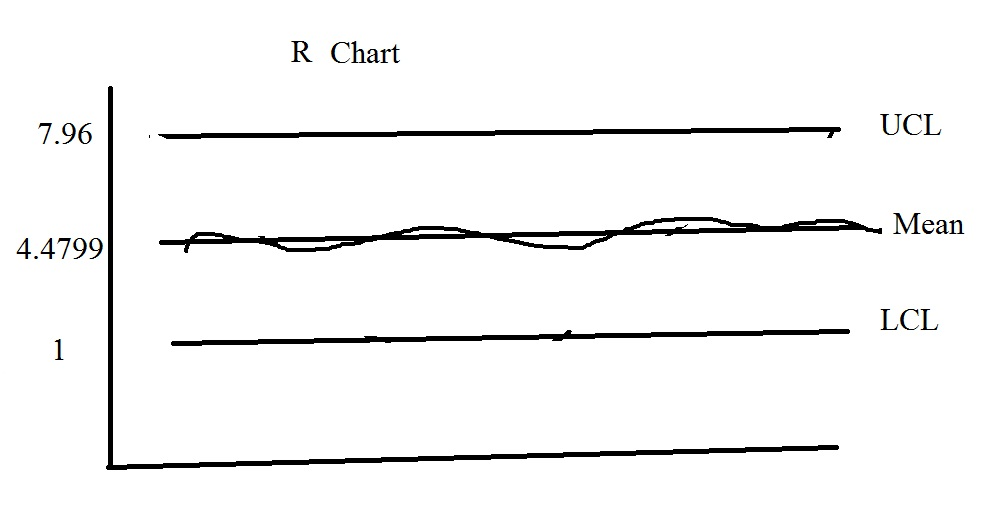# Question & Answer: Auto pistons at Wemming Chung's plant in Shanghai are produced in a forging…..Auto pistons at Wemming Chung’s plant in Shanghai are produced in a forging process, and the diameter is a critical factor that must be controlled. From sample sizes of 10 pistons produced each day, the mean and the range of this diameter have been as follows: a) What is the value of x? b) What is the value of R bar? c) What are the UCL_x bar and LCL_x bar, using 3 sigma? Plot the data. d) What are the UCL_R and LCL_R, using 3 sigma? Plot the data. e) If the true diameter mean should be 155 mm and you want this as your center (nominal) line, what are the new UCL_x bar and LCL_x bar?

x double bar = mean of means = mean or average of (day 1 to day 5 means in MM ) = average of (156.9, 153.2, etc, 156.6)

Don't use plagiarized sources. Get Your Custom Essay on
Question & Answer: Auto pistons at Wemming Chung's plant in Shanghai are produced in a forging…..
GET AN ESSAY WRITTEN FOR YOU FROM AS LOW AS \$13/PAGE
 x 156.9 153.2 153.6 155.5 156.6 Mean = Average = 155.16

a) x double bar = 155.16 mm

Similarly, add up all the range values and divide by 5 to find the arithmetic mean or average:

Excel Function: Average(values)

 Range 4.2 4.6 4.1 5 4.5 (4.2+4.6+4.1+5+4.5)/5 = 4.48

b) R bar = 4.4799999999 mm

c)

X:

The control chart built in value A 2 = 0.307999999 for 10 piston samples

Upper Control Limit UCL x bar = average + A 2 R = 155.16 + (0.30799999999 * 4.479999999999) = 156.54 mm = 157 mm (approximately(approx))

Lower Control Limit LCL x bar = average – A 2 R = 155.16 – (0.30799999999 * 4.479999999999) = 153.779999999mm = 154 mm (Approx)

d)

R:

R is already found to be R = 4.479999999999999999

The standard tables give D4 = 1.777 and D3 = 0.223

UCL R = D 4 * R = 1.777 * 4.479999999999999999 = 7.961 mm

LCL R = D 3 * R = 0.223 * 4.479999999999999999 = 0.999 = 1 mm

e)

new mean = 155

new UCL x = 155 + (0.30799999999 * 4.479999999999) = 156.37999999 mm

new LCL x = 155 – (0.30799999999 * 4.479999999999) = 153.6202 mm

Charts:

X:R: